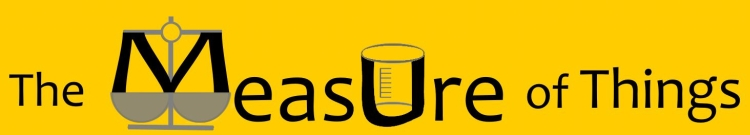Bluebulb Projects presents:Enter a measurement to see comparisons

Equivalents in other units

How big is 51 squares?

Sort Order:
Closest first | Highest first | Lowest first

 It's about 700,000,000 times as big as a Ballpoint Pen Tip. In other words, 51 squares is 680,000,000 times the size of a Ballpoint Pen Tip, and the size of a Ballpoint Pen Tip is 0.00000000150 times that amount. (a.k.a. Biro, a.k.a. ball pen, a.k.a. dot pen) (for medium tip) The most common size of ballpoint pen â€” a medium-tip â€” measures 0.0000000750 squares. A typical ballpoint pen can write more than 8,500 m (28,000 ft) before running out of ink. It's about 6,000,000 times as big as a Nailhead. In other words, 51 squares is 6,095,000 times the size of a Nailhead, and the size of a Nailhead is 0.00000016410 times that amount. (for 11-gauge wire nai) An 11-gauge nailhead measures 0.0000083680 squares. Nails have been used since ancient times; archaeologists have discovered Ancient Egyptian nails that are over 5,000 years old. It's about 2,000,000 times as big as a Dime. In other words, 51 squares is 1,881,000 times the size of a Dime, and the size of a Dime is 0.00000053160 times that amount. (United States ten-cent coin) (a.k.a. Roosevelt dime)The dime, in its current design since 1946, has a diameter of 17.91 mm (0.705 in) and an area of 0.000027110 squares. It's about 1,500,000 times as big as a Penny. In other words, 51 squares is 1,660,000 times the size of a Penny, and the size of a Penny is 0.0000006020 times that amount. (United States one-cent coin) (a.k.a. Lincoln penny, a.k.a. Cent, a.k.a. One cent piece)The Lincoln penny, in its current design since 1909, has a diameter of 19.05 mm (0.75 in) and an area of 0.00003070 squares. It's about 1,500,000 times as big as a Nickel. In other words, 51 squares is 1,341,000 times the size of a Nickel, and the size of a Nickel is 0.00000074570 times that amount. (United States five-cent coin) (a.k.a. Jefferson nickel)The nickel, in its current design since 1938, has a diameter of 21.21 mm (0.835 in) and an area of 0.000038030 squares. It's about 1,000,000 times as big as a Quarter. In other words, 51 squares is 1,025,000 times the size of a Quarter, and the size of a Quarter is 0.00000097560 times that amount. (United States quarter-dollar coin) (a.k.a. Quarter dollar, a.k.a. Washington quarter, a.k.a. two bits)The George Washington quarter, in circulation in various forms since 1932, has a diameter of 24.26 mm (0.955 in) and an area of 0.000049750 squares. It's about 1,000,000 times as big as a Postage Stamp. In other words, the size of a Postage Stamp is 0.000001000 times 51 squares. (average; United States dimensions)A typical postage stamp measures an average of 0.0000400 squares. One of the most prized stamps among collectors is the US-issued "Inverted Jenny" stamp printed in 1918, which features an upside-down image of Curtiss JN-4 ("Jenny") airplane; a single stamp sold for \$977,500 at a 2007 auction.